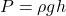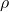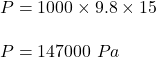## What gauge pressure is required in the city water mains for a stream from a fire hose connected to the mains to reach a vertical height of 1

Question

What gauge pressure is required in the city water mains for a stream from a fire hose connected to the mains to reach a vertical height of 15.0 m

in progress 0
6 months 2021-07-12T11:10:28+00:00 2 Answers 35 views 0

## Answers ( )

The gauge pressure is 147000 Pa.

Explanation:

Height, h = 15 m

density of water, d= 1000 kg/m^3

gravity, g = 9.8 m/s^2

The gauge pressure is the pressure exerted by the fluid.

The pressure exerted by the fluid is given by

P  = h d g

P = 15 x 1000 x 9.8 =  147000 Pa

The gauge pressure is equal to 147 kPa.

Explanation:

The pressure exerted by fluid is given by :Whereis density of water

h is height

So, put all the values,or

P = 147 kPa

So, the gauge pressure is equal to 147 kPa.